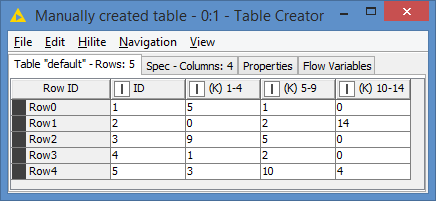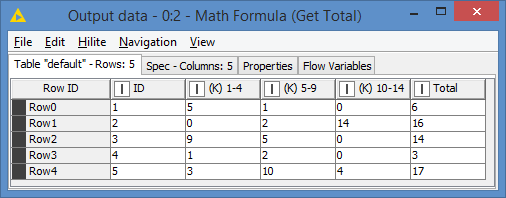# Math Formula - Column Problem

Hello everyone,I hope that all of you are well.

I need some help in the Math Formula section of Knime.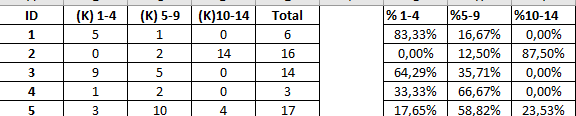I tried to do(percentages) this Excel in Knime with Math Formula and Math Formula(Multi Column). However, Knime produced only one column.

Is there any solution about it?

Best Regards,

Aldwyn

Welcome to the forum, @Aldwyn.

How are you configuring the Math Formula (Multi Column) node? When I do it I get 3 columns.

1 Like

Hi @Aldwyn ,
Your Multi Column Math node should be formatted as follows:

Result: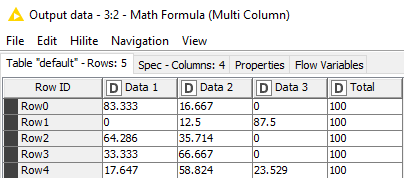Use the “Column Filter” node to remove the “Total” column if you don’t want it.

If you don’t have the “Total” column, you can use the “Column Aggregator” node to sum that up, prior to the Multi Column Math node.

2 Likes

@Snowy provided a detailed and great response.
Is the total column already available (which would be assumed based on your screenshot) or do you need to calc it?
br

Hi @Snowy , you can also exclude the column Total from your list:

Yes, but that doesn’t actually remove it from the resulting table set, it just doesn’t apply the calculation to it…

2 Likes

Hi @Snowy , yes I was looking at it from the calculation perspectiveThe column does not need to be calculated, as it will always be 100%, and it was not part of the 3 columns that @Aldwyn wanted to transform. However, I do understand why you added it, because in your example, you are replacing the original columns, and that would avoid any confusion.

I’ve put something together that does not replace the original values as it is often the case that people want to keep both the numbers and the percentage, and it is how @Aldwyn presented the expected results, although that could simply because it was done in Excel.

Hi @Aldwyn , and welcome to the Knime Community. This is how my workflow looks like: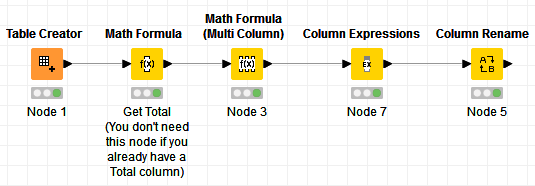As raised by some, may be the Total column is provided, or may be it’s not. I added a node that generates the Total column for you. If you already have the Total column, you can just remove that node.

Input data: